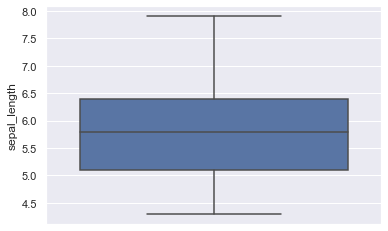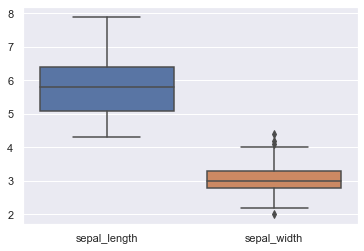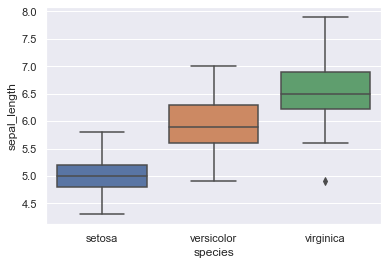## One single numerical variable

The simplest form of boxplot: analysis of the overall distribution of a single numerical variable in an entire dataset.

``````# libraries & dataset
import seaborn as sns
import matplotlib.pyplot as plt
# set a grey background (use sns.set_theme() if seaborn version 0.11.0 or above)
sns.set(style="darkgrid")

sns.boxplot(y=df["sepal_length"])
plt.show()``````## Several numerical variables

If you intend to add more information in a single figure, you can also visualize several numerical variables distributions by setting the `data` argument and specifying a dataset with several numerical columns.

``````# libraries & dataset
import seaborn as sns
import matplotlib.pyplot as plt
# set a grey background (use sns.set_theme() if seaborn version 0.11.0 or above)
sns.set(style="darkgrid")

sns.boxplot(data=df.loc[:, ['sepal_length', 'sepal_width']])
plt.show()``````## One numerical variable and several groups

Depending on your data, you may want to have a better understanding of the distribution of a given variable between two or more groups. You can do so by specifying the 'x' parameter in the boxplot() function.

``````# libraries & dataset
import seaborn as sns
import matplotlib.pyplot as plt
# set a grey background (use sns.set_theme() if seaborn version 0.11.0 or above)
sns.set(style="darkgrid")